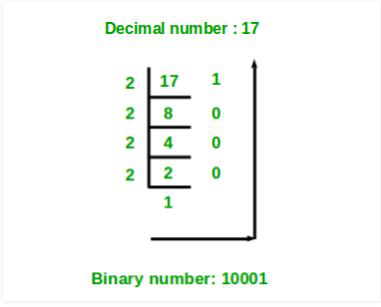GeeksforGeeks App
Open AppBrowser
Continue

# Java Program to Convert a Decimal Number to Binary Number using Arrays as Stacks

Given an Integer number convert into Binary Number using arrays as a stack.

Example:

```Input : 10
Output: 1010
Input : 16
Output: 10000```

Approach:

1. Divide the number by 2 and store the remainder of the number in the array.
2. Divide the number by 2.
3. Repeat the process until the number becomes zero.
4. Print the array in reverse order.## Java

 `// Java Program to Convert a Decimal Number``// to Binary Number using Arrays as Stacks` `import` `java.util.*;``public` `class` `DecimalToBinary {``    ``static` `int` `arr[] = ``new` `int``[``1000``];` `    ``// maintaining count variable``    ``// as the top of the stack``    ``static` `int` `count;` `    ``// push at the count index and increment the count``    ``public` `static` `void` `push(``int` `n) {``      ``arr[count++] = n;``    ``}` `    ``// pop all the elements starting``    ``// from count-1 till 0``    ``public` `static` `void` `pop()``    ``{``        ``for` `(``int` `i = count - ``1``; i >= ``0``; i--) {``            ``System.out.print(arr[i]);``        ``}``    ``}` `    ``public` `static` `void` `main(String args[])``    ``{``        ``int` `num = ``46``;` `        ``while` `(num > ``0``) {``            ``int` `r = num % ``2``;``            ``push(r);``            ``num /= ``2``;``        ``}` `        ``System.out.print(``"Binary equivalent: "``);` `        ``pop();``    ``}``}`

Output

`Binary equivalent: 101110`

Time complexity: O(logn) for given input number n

My Personal Notes arrow_drop_up